# forecast

Forecast vector error-correction (VEC) model responses

## Syntax

``Y = forecast(Mdl,numperiods,Y0)``
``Y = forecast(Mdl,numperiods,Y0,Name,Value)``
``````[Y,YMSE] = forecast(___)``````

## Description

example

````Y = forecast(Mdl,numperiods,Y0)` returns a path of minimum mean squared error (MMSE) forecasts (`Y`) over the length `numperiods` forecast horizon using the fully specified VEC(p – 1) model `Mdl`. The forecasted responses represent the continuation of the presample data `Y0`.```

example

````Y = forecast(Mdl,numperiods,Y0,Name,Value)` uses additional options specified by one or more name-value arguments. For example, `'X',X,'YF',YF` specifies `X` as future exogenous predictor data for the regression component and `YF` as future response data for conditional forecasting.```

example

``````[Y,YMSE] = forecast(___)``` returns the corresponding mean squared error (MSE) of each forecasted response using any of the input arguments in the previous syntaxes.```

## Examples

collapse all

Consider a VEC model for the following seven macroeconomic series. Then, fit the model to the data and forecast responses 12 quarters into the future.

• Gross domestic product (GDP)

• GDP implicit price deflator

• Paid compensation of employees

• Nonfarm business sector hours of all persons

• Effective federal funds rate

• Personal consumption expenditures

• Gross private domestic investment

Suppose that a cointegrating rank of 4 and one short-run term are appropriate, that is, consider a VEC(1) model.

Load the `Data_USEconVECModel` data set.

`load Data_USEconVECModel`

For more information on the data set and variables, enter `Description` at the command line.

Determine whether the data needs to be preprocessed by plotting the series on separate plots.

```figure; subplot(2,2,1) plot(FRED.Time,FRED.GDP); title('Gross Domestic Product'); ylabel('Index'); xlabel('Date'); subplot(2,2,2) plot(FRED.Time,FRED.GDPDEF); title('GDP Deflator'); ylabel('Index'); xlabel('Date'); subplot(2,2,3) plot(FRED.Time,FRED.COE); title('Paid Compensation of Employees'); ylabel('Billions of \$'); xlabel('Date'); subplot(2,2,4) plot(FRED.Time,FRED.HOANBS); title('Nonfarm Business Sector Hours'); ylabel('Index'); xlabel('Date');```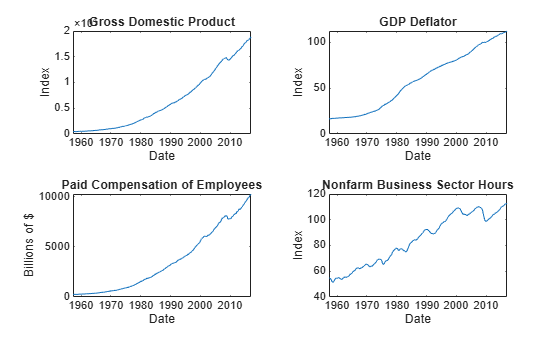```figure; subplot(2,2,1) plot(FRED.Time,FRED.FEDFUNDS); title('Federal Funds Rate'); ylabel('Percent'); xlabel('Date'); subplot(2,2,2) plot(FRED.Time,FRED.PCEC); title('Consumption Expenditures'); ylabel('Billions of \$'); xlabel('Date'); subplot(2,2,3) plot(FRED.Time,FRED.GPDI); title('Gross Private Domestic Investment'); ylabel('Billions of \$'); xlabel('Date');```Stabilize all series, except the federal funds rate, by applying the log transform. Scale the resulting series by 100 so that all series are on the same scale.

```FRED.GDP = 100*log(FRED.GDP); FRED.GDPDEF = 100*log(FRED.GDPDEF); FRED.COE = 100*log(FRED.COE); FRED.HOANBS = 100*log(FRED.HOANBS); FRED.PCEC = 100*log(FRED.PCEC); FRED.GPDI = 100*log(FRED.GPDI);```

Create a VEC(1) model using the shorthand syntax. Specify the variable names.

```Mdl = vecm(7,4,1); Mdl.SeriesNames = FRED.Properties.VariableNames;```

`Mdl` is a `vecm` model object. All properties containing `NaN` values correspond to parameters to be estimated given data.

Estimate the model using the entire data set and the default options.

`EstMdl = estimate(Mdl,FRED.Variables)`
```EstMdl = vecm with properties: Description: "7-Dimensional Rank = 4 VEC(1) Model" SeriesNames: "GDP" "GDPDEF" "COE" ... and 4 more NumSeries: 7 Rank: 4 P: 2 Constant: [14.1329 8.77841 -7.20359 ... and 4 more]' Adjustment: [7×4 matrix] Cointegration: [7×4 matrix] Impact: [7×7 matrix] CointegrationConstant: [-28.6082 109.555 -77.0912 ... and 1 more]' CointegrationTrend: [4×1 vector of zeros] ShortRun: {7×7 matrix} at lag  Trend: [7×1 vector of zeros] Beta: [7×0 matrix] Covariance: [7×7 matrix] ```

`EstMdl` is an estimated `vecm` model object. It is fully specified because all parameters have known values. By default, `estimate` imposes the constraints of the H1 Johansen VEC model form by removing the cointegrating trend and linear trend terms from the model. Parameter exclusion from estimation is equivalent to imposing equality constraints to zero.

Forecast responses from the estimated model over a three-year horizon. Specify the entire data set as presample observations.

```numperiods = 12; Y0 = FRED.Variables; Y = forecast(EstMdl,numperiods,Y0);```

`Y` is a 12-by-7 matrix of forecasted responses. Rows correspond to the forecast horizon, and columns correspond to the variables in `EstMdl.SeriesNames`.

Plot the forecasted responses and the last 50 true responses.

```fh = dateshift(FRED.Time(end),'end','quarter',1:12); figure; subplot(2,2,1) h1 = plot(FRED.Time((end-49):end),FRED.GDP((end-49):end)); hold on h2 = plot(fh,Y(:,1)); title('Gross Domestic Product'); ylabel('Index (scaled)'); xlabel('Date'); h = gca; fill([FRED.Time(end) fh([end end]) FRED.Time(end)],h.YLim([1 1 2 2]),'k',... 'FaceAlpha',0.1,'EdgeColor','none'); legend([h1 h2],'True','Forecast','Location','Best') hold off subplot(2,2,2) h1 = plot(FRED.Time((end-49):end),FRED.GDPDEF((end-49):end)); hold on h2 = plot(fh,Y(:,2)); title('GDP Deflator'); ylabel('Index (scaled)'); xlabel('Date'); h = gca; fill([FRED.Time(end) fh([end end]) FRED.Time(end)],h.YLim([1 1 2 2]),'k',... 'FaceAlpha',0.1,'EdgeColor','none'); legend([h1 h2],'True','Forecast','Location','Best') hold off subplot(2,2,3) h1 = plot(FRED.Time((end-49):end),FRED.COE((end-49):end)); hold on h2 = plot(fh,Y(:,3)); title('Paid Compensation of Employees'); ylabel('Billions of \$ (scaled)'); xlabel('Date'); h = gca; fill([FRED.Time(end) fh([end end]) FRED.Time(end)],h.YLim([1 1 2 2]),'k',... 'FaceAlpha',0.1,'EdgeColor','none'); legend([h1 h2],'True','Forecast','Location','Best') hold off subplot(2,2,4) h1 = plot(FRED.Time((end-49):end),FRED.HOANBS((end-49):end)); hold on h2 = plot(fh,Y(:,4)); title('Nonfarm Business Sector Hours'); ylabel('Index (scaled)'); xlabel('Date'); h = gca; fill([FRED.Time(end) fh([end end]) FRED.Time(end)],h.YLim([1 1 2 2]),'k',... 'FaceAlpha',0.1,'EdgeColor','none'); legend([h1 h2],'True','Forecast','Location','Best') hold off```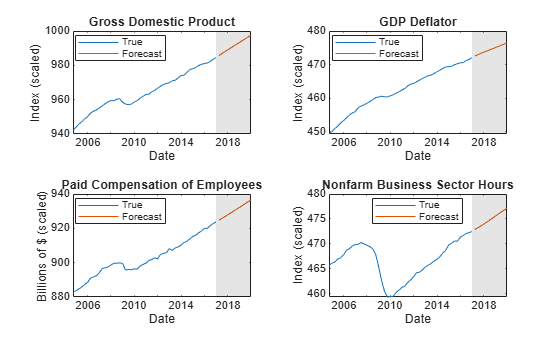```figure; subplot(2,2,1) h1 = plot(FRED.Time((end-49):end),FRED.FEDFUNDS((end-49):end)); hold on h2 = plot(fh,Y(:,5)); title('Federal Funds Rate'); ylabel('Percent'); xlabel('Date'); h = gca; fill([FRED.Time(end) fh([end end]) FRED.Time(end)],h.YLim([1 1 2 2]),'k',... 'FaceAlpha',0.1,'EdgeColor','none'); legend([h1 h2],'True','Forecast','Location','Best') hold off subplot(2,2,2) h1 = plot(FRED.Time((end-49):end),FRED.PCEC((end-49):end)); hold on h2 = plot(fh,Y(:,6)); title('Consumption Expenditures'); ylabel('Billions of \$ (scaled)'); xlabel('Date'); h = gca; fill([FRED.Time(end) fh([end end]) FRED.Time(end)],h.YLim([1 1 2 2]),'k',... 'FaceAlpha',0.1,'EdgeColor','none'); legend([h1 h2],'True','Forecast','Location','Best') hold off subplot(2,2,3) h1 = plot(FRED.Time((end-49):end),FRED.GPDI((end-49):end)); hold on h2 = plot(fh,Y(:,7)); title('Gross Private Domestic Investment'); ylabel('Billions of \$ (scaled)'); xlabel('Date'); h = gca; fill([FRED.Time(end) fh([end end]) FRED.Time(end)],h.YLim([1 1 2 2]),'k',... 'FaceAlpha',0.1,'EdgeColor','none'); legend([h1 h2],'True','Forecast','Location','Best') hold off```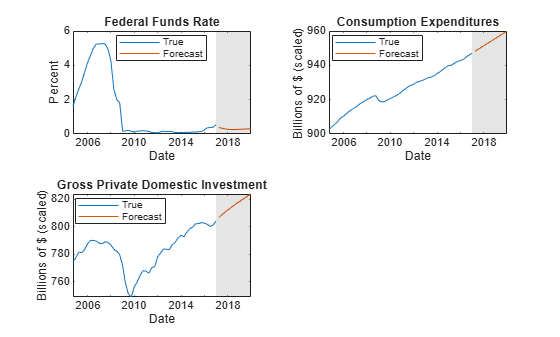Consider the model and data in Forecast Unconditional Response Series from VEC Model.

Load the `Data_USEconVECModel` data set and preprocess the data.

```load Data_USEconVECModel FRED.GDP = 100*log(FRED.GDP); FRED.GDPDEF = 100*log(FRED.GDPDEF); FRED.COE = 100*log(FRED.COE); FRED.HOANBS = 100*log(FRED.HOANBS); FRED.PCEC = 100*log(FRED.PCEC); FRED.GPDI = 100*log(FRED.GPDI);```

The `Data_Recessions` data set contains the beginning and ending serial dates of recessions. Load the data set. Convert the matrix of date serial numbers to a datetime array.

```load Data_Recessions dtrec = datetime(Recessions,'ConvertFrom','datenum');```

Create a dummy variable that identifies periods in which the U.S. was in a recession or worse. Specifically, the variable should be `1` if `FRED.Time` occurs during a recession, and `0` otherwise.

```isin = @(x)(any(dtrec(:,1) <= x & x <= dtrec(:,2))); isrecession = double(arrayfun(isin,FRED.Time));```

Create a VEC(1) model using the shorthand syntax. Assume that the appropriate cointegration rank is 4. You do not have to specify the presence of a regression component when creating the model. Specify the variable names.

```Mdl = vecm(7,4,1); Mdl.SeriesNames = FRED.Properties.VariableNames;```

Estimate the model using all but the last three years of data. Specify the predictor identifying whether the observation was measured during a recession.

```bfh = FRED.Time(end) - years(3); estIdx = FRED.Time < bfh; EstMdl = estimate(Mdl,FRED{estIdx,:},'X',isrecession(estIdx));```

Forecast a path of quarterly responses three years into the future.

```Y0 = FRED{estIdx,:}; Y = forecast(EstMdl,12,Y0,'X',isrecession(~estIdx));```

`Y` is a 12-by-7 matrix of simulated responses. Rows correspond to the forecast horizon, and columns correspond to the variables in `EstMdl.SeriesNames`.

Plot the forecasted responses and the last 40 true responses.

```figure; subplot(2,2,1) h1 = plot(FRED.Time((end-39):end),FRED.GDP((end-39):end)); hold on h2 = plot(FRED.Time(~estIdx),Y(:,1)); title('Gross Domestic Product'); ylabel('Index (scaled)'); xlabel('Date'); h = gca; fill([bfh h.XLim([2 2]) bfh],h.YLim([1 1 2 2]),'k',... 'FaceAlpha',0.1,'EdgeColor','none'); legend([h1 h2],'True','Forecast','Location','Best') hold off subplot(2,2,2) h1 = plot(FRED.Time((end-39):end),FRED.GDPDEF((end-39):end)); hold on h2 = plot(FRED.Time(~estIdx),Y(:,2)); title('GDP Deflator'); ylabel('Index (scaled)'); xlabel('Date'); h = gca; fill([bfh h.XLim([2 2]) bfh],h.YLim([1 1 2 2]),'k',... 'FaceAlpha',0.1,'EdgeColor','none'); legend([h1 h2],'True','Forecast','Location','Best') hold off subplot(2,2,3) h1 = plot(FRED.Time((end-39):end),FRED.COE((end-39):end)); hold on h2 = plot(FRED.Time(~estIdx),Y(:,3)); title('Paid Compensation of Employees'); ylabel('Billions of \$ (scaled)'); xlabel('Date'); h = gca; fill([bfh h.XLim([2 2]) bfh],h.YLim([1 1 2 2]),'k',... 'FaceAlpha',0.1,'EdgeColor','none'); legend([h1 h2],'True','Forecast','Location','Best') hold off subplot(2,2,4) h1 = plot(FRED.Time((end-39):end),FRED.HOANBS((end-39):end)); hold on h2 = plot(FRED.Time(~estIdx),Y(:,4)); title('Nonfarm Business Sector Hours'); ylabel('Index (scaled)'); xlabel('Date'); h = gca; fill([bfh h.XLim([2 2]) bfh],h.YLim([1 1 2 2]),'k',... 'FaceAlpha',0.1,'EdgeColor','none'); legend([h1 h2],'True','Forecast','Location','Best') hold off```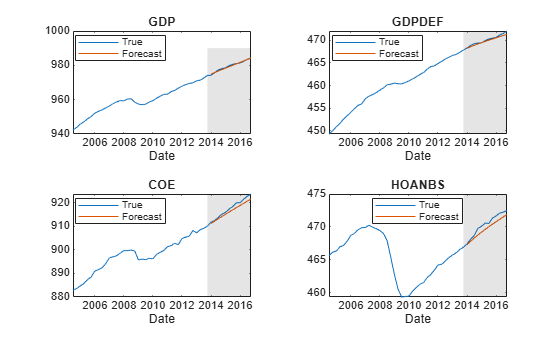```figure; subplot(2,2,1) h1 = plot(FRED.Time((end-39):end),FRED.FEDFUNDS((end-39):end)); hold on h2 = plot(FRED.Time(~estIdx),Y(:,5)); title('Federal Funds Rate'); ylabel('Percent'); xlabel('Date'); h = gca; fill([bfh h.XLim([2 2]) bfh],h.YLim([1 1 2 2]),'k',... 'FaceAlpha',0.1,'EdgeColor','none'); legend([h1 h2],'True','Forecast','Location','Best') hold off subplot(2,2,2) h1 = plot(FRED.Time((end-39):end),FRED.PCEC((end-39):end)); hold on h2 = plot(FRED.Time(~estIdx),Y(:,6)); title('Consumption Expenditures'); ylabel('Billions of \$ (scaled)'); xlabel('Date'); h = gca; fill([bfh h.XLim([2 2]) bfh],h.YLim([1 1 2 2]),'k',... 'FaceAlpha',0.1,'EdgeColor','none'); legend([h1 h2],'True','Forecast','Location','Best') hold off subplot(2,2,3) h1 = plot(FRED.Time((end-39):end),FRED.GPDI((end-39):end)); hold on h2 = plot(FRED.Time(~estIdx),Y(:,7)); title('Gross Private Domestic Investment'); ylabel('Billions of \$ (scaled)'); xlabel('Date'); h = gca; fill([bfh h.XLim([2 2]) bfh],h.YLim([1 1 2 2]),'k',... 'FaceAlpha',0.1,'EdgeColor','none'); legend([h1 h2],'True','Forecast','Location','Best') hold off```Analyze forecast accuracy using forecast intervals over a three-year horizon. This example follows from Forecast Unconditional Response Series from VEC Model.

Load the `Data_USEconVECModel` data set and preprocess the data.

```load Data_USEconVECModel FRED.GDP = 100*log(FRED.GDP); FRED.GDPDEF = 100*log(FRED.GDPDEF); FRED.COE = 100*log(FRED.COE); FRED.HOANBS = 100*log(FRED.HOANBS); FRED.PCEC = 100*log(FRED.PCEC); FRED.GPDI = 100*log(FRED.GPDI);```

Estimate a VEC(1) model. Reserve the last three years of data to assess forecast accuracy. Assume that the appropriate cointegration rank is 4, and the H1 Johansen form is appropriate for the model.

```bfh = FRED.Time(end) - years(3); estIdx = FRED.Time < bfh; Mdl = vecm(7,4,1); Mdl.SeriesNames = FRED.Properties.VariableNames; EstMdl = estimate(Mdl,FRED{estIdx,:});```

Forecast responses from the estimated model over a three-year horizon. Specify all in-sample observations as a presample. Return the MSE of the forecasts.

```numperiods = 12; Y0 = FRED{estIdx,:}; [Y,YMSE] = forecast(EstMdl,numperiods,Y0);```

`Y` is a 12-by-7 matrix of forecasted responses. `YMSE` is a 12-by-1 cell vector of 7-by-7 matrices corresponding to the MSEs.

Extract the main diagonal elements from the matrices in each cell of `YMSE`. Apply the square root of the result to obtain standard errors.

```extractMSE = @(x)diag(x)'; MSE = cellfun(extractMSE,YMSE,'UniformOutput',false); SE = sqrt(cell2mat(MSE));```

Estimate approximate 95% forecast intervals for each response series.

```YFI = zeros(numperiods,Mdl.NumSeries,2); YFI(:,:,1) = Y - 2*SE; YFI(:,:,2) = Y + 2*SE;```

Plot the forecasted responses and the last 40 true responses.

```figure; subplot(2,2,1) h1 = plot(FRED.Time((end-39):end),FRED.GDP((end-39):end)); hold on h2 = plot(FRED.Time(~estIdx),Y(:,1)); h3 = plot(FRED.Time(~estIdx),YFI(:,1,1),'k--'); plot(FRED.Time(~estIdx),YFI(:,1,2),'k--'); title('Gross Domestic Product'); ylabel('Index (scaled)'); xlabel('Date'); h = gca; fill([bfh h.XLim([2 2]) bfh],h.YLim([1 1 2 2]),'k',... 'FaceAlpha',0.1,'EdgeColor','none'); legend([h1 h2 h3],'True','Forecast','95% Forecast interval',... 'Location','best'); hold off subplot(2,2,2) h1 = plot(FRED.Time((end-39):end),FRED.GDPDEF((end-39):end)); hold on h2 = plot(FRED.Time(~estIdx),Y(:,2)); h3 = plot(FRED.Time(~estIdx),YFI(:,2,1),'k--'); plot(FRED.Time(~estIdx),YFI(:,2,2),'k--'); title('GDP Deflator'); ylabel('Index (scaled)'); xlabel('Date'); h = gca; fill([bfh h.XLim([2 2]) bfh],h.YLim([1 1 2 2]),'k',... 'FaceAlpha',0.1,'EdgeColor','none'); legend([h1 h2 h3],'True','Forecast','95% Forecast interval',... 'Location','best'); hold off subplot(2,2,3) h1 = plot(FRED.Time((end-39):end),FRED.COE((end-39):end)); hold on h2 = plot(FRED.Time(~estIdx),Y(:,3)); h3 = plot(FRED.Time(~estIdx),YFI(:,3,1),'k--'); plot(FRED.Time(~estIdx),YFI(:,3,2),'k--'); title('Paid Compensation of Employees'); ylabel('Billions of \$ (scaled)'); xlabel('Date'); h = gca; fill([bfh h.XLim([2 2]) bfh],h.YLim([1 1 2 2]),'k',... 'FaceAlpha',0.1,'EdgeColor','none'); legend([h1 h2 h3],'True','Forecast','95% Forecast interval',... 'Location','best'); hold off subplot(2,2,4) h1 = plot(FRED.Time((end-39):end),FRED.HOANBS((end-39):end)); hold on h2 = plot(FRED.Time(~estIdx),Y(:,4)); h3 = plot(FRED.Time(~estIdx),YFI(:,4,1),'k--'); plot(FRED.Time(~estIdx),YFI(:,4,2),'k--'); title('Nonfarm Business Sector Hours'); ylabel('Index (scaled)'); xlabel('Date'); h = gca; fill([bfh h.XLim([2 2]) bfh],h.YLim([1 1 2 2]),'k',... 'FaceAlpha',0.1,'EdgeColor','none'); legend([h1 h2 h3],'True','Forecast','95% Forecast interval',... 'Location','best'); hold off```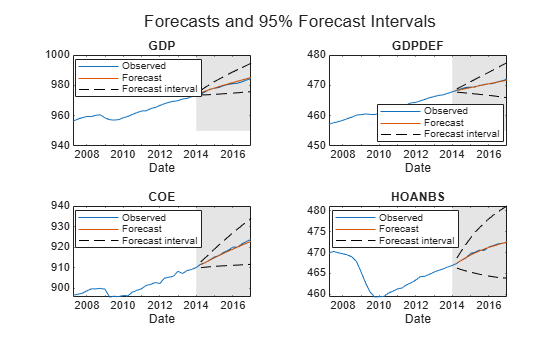```figure; subplot(2,2,1) h1 = plot(FRED.Time((end-39):end),FRED.FEDFUNDS((end-39):end)); hold on h2 = plot(FRED.Time(~estIdx),Y(:,5)); h3 = plot(FRED.Time(~estIdx),YFI(:,5,1),'k--'); plot(FRED.Time(~estIdx),YFI(:,5,2),'k--'); title('Federal Funds Rate'); ylabel('Percent'); xlabel('Date'); h = gca; fill([bfh h.XLim([2 2]) bfh],h.YLim([1 1 2 2]),'k',... 'FaceAlpha',0.1,'EdgeColor','none'); legend([h1 h2 h3],'True','Forecast','95% Forecast interval',... 'Location','best'); hold off subplot(2,2,2) h1 = plot(FRED.Time((end-39):end),FRED.PCEC((end-39):end)); hold on h2 = plot(FRED.Time(~estIdx),Y(:,6)); h3 = plot(FRED.Time(~estIdx),YFI(:,6,1),'k--'); plot(FRED.Time(~estIdx),YFI(:,6,2),'k--'); title('Consumption Expenditures'); ylabel('Billions of \$ (scaled)'); xlabel('Date'); h = gca; fill([bfh h.XLim([2 2]) bfh],h.YLim([1 1 2 2]),'k',... 'FaceAlpha',0.1,'EdgeColor','none'); legend([h1 h2 h3],'True','Forecast','95% Forecast interval',... 'Location','best'); hold off subplot(2,2,3) h1 = plot(FRED.Time((end-39):end),FRED.GPDI((end-39):end)); hold on h2 = plot(FRED.Time(~estIdx),Y(:,7)); h3 = plot(FRED.Time(~estIdx),YFI(:,7,1),'k--'); plot(FRED.Time(~estIdx),YFI(:,7,2),'k--'); title('Gross Private Domestic Investment'); ylabel('Billions of \$ (scaled)'); xlabel('Date'); h = gca; fill([bfh h.XLim([2 2]) bfh],h.YLim([1 1 2 2]),'k',... 'FaceAlpha',0.1,'EdgeColor','none'); legend([h1 h2 h3],'True','Forecast','95% Forecast interval',... 'Location','best'); hold off```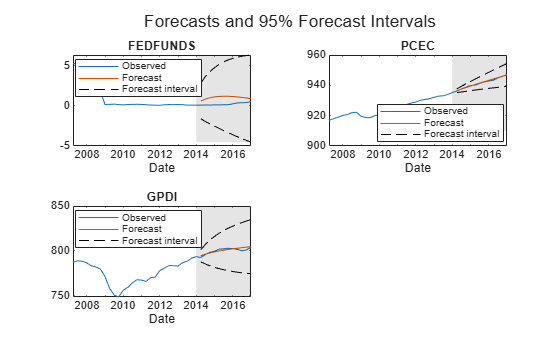## Input Arguments

collapse all

VEC model, specified as a `vecm` model object created by `vecm` or `estimate`. `Mdl` must be fully specified.

Forecast horizon, or the number of time points in the forecast period, specified as a positive integer.

Data Types: `double`

Presample responses that provide initial values for the forecasts, specified as a `numpreobs`-by-`numseries` numeric matrix or a `numpreobs`-by-`numseries`-by-`numprepaths` numeric array.

`numpreobs` is the number of presample observations. `numseries` is the number of response series (`Mdl.NumSeries`). `numprepaths` is the number of presample response paths.

Rows correspond to presample observations, and the last row contains the latest observation. `Y0` must contain at least `Mdl.P` rows. If you supply more rows than necessary, `forecast` uses only the latest `Mdl.P` observations.

Columns must correspond to the response series names in `Mdl.SeriesNames`.

Pages correspond to separate, independent paths.

• If you do not specify the `YF` name-value pair argument, then `forecast` initializes each forecasted path (page) using the corresponding page of `Y0`. Therefore, the output argument `Y` has `numprepaths` pages.

• If you specify the `YF` name-value pair argument, then `forecast` takes one of these actions.

• If `Y0` is a matrix, then `forecast` initializes each forecast path (page) in `YF` using `Y0`. Therefore, all paths in the output argument `Y` derive from common initial conditions.

• Otherwise, `forecast` applies `Y0(:,:,j)` to initialize forecasting path `j`. `Y0` must have at least `numpaths` pages, and `forecast` uses only the first `numpaths` pages.

Among all pages, observations in a particular row occur at the same time.

Data Types: `double`

### Name-Value Arguments

Specify optional pairs of arguments as `Name1=Value1,...,NameN=ValueN`, where `Name` is the argument name and `Value` is the corresponding value. Name-value arguments must appear after other arguments, but the order of the pairs does not matter.

Before R2021a, use commas to separate each name and value, and enclose `Name` in quotes.

Example: `'X',X,'YF',YF` uses the matrix `X` as predictor data in the regression component, and the matrix `YF` as partially known future responses for conditional forecasting.

Forecasted time series of predictors to include in the model regression component, specified as the comma-separated pair consisting of `'X'` and a numeric matrix containing `numpreds` columns.

`numpreds` is the number of predictor variables (`size(Mdl.Beta,2)`).

Rows correspond to observations. Row `j` contains the `j`-step-ahead forecast. `X` must have at least `numperiods` rows. If you supply more rows than necessary, `forecast` uses only the earliest `numperiods` observations. The first row contains the earliest observation.

Columns correspond to individual predictor variables. All predictor variables are present in the regression component of each response equation.

`forecast` applies `X` to each path (page); that is, `X` represents one path of observed predictors.

To maintain model consistency into the forecast horizon, it is a good practice to specify forecasted predictors when `Mdl` has a regression component.

By default, `forecast` excludes the regression component, regardless of its presence in `Mdl`.

Data Types: `double`

Future multivariate response series for conditional forecasting, specified as the comma-separated pair consisting of `'YF'` and a numeric matrix or 3-D array containing `numseries` columns.

Rows correspond to observations in the forecast horizon, and the first row is the earliest observation. Specifically, row `j` in sample path `k` (`YF(j,:,k)`) contains the responses `j` periods into the future. `YF` must have at least `numperiods` rows to cover the forecast horizon. If you supply more rows than necessary, `forecast` uses only the first `numperiods` rows.

Columns correspond to the response variables in `Y0`.

Pages correspond to sample paths. Specifically, path `k` (`YF(:,:,k)`) captures the state, or knowledge, of the response series as they evolve from the presample past (`Y0`) into the future.

• If `YF` is a matrix, then `forecast` applies `YF` to each of the `numpaths` output paths (see `Y0`).

• Otherwise, `YF` must have at least `numpaths` pages. If you supply more pages than necessary, `forecast` uses only the first `numpaths` pages.

Elements of `YF` can be numeric scalars or missing values (indicated by `NaN` values). `forecast` treats numeric scalars as deterministic future responses that are known in advance, for example, set by policy. `forecast` forecasts responses for corresponding `NaN` values conditional on the known values.

By default, `YF` is an array composed of `NaN` values indicating a complete lack of knowledge of the future state of all responses in the forecast horizon. In this case, `forecast` estimates conventional MMSE forecasts.

For more details, see Algorithms.

Example: Consider forecasting one path of a VEC model composed of four response series three periods into the future. Suppose that you have prior knowledge about some of the future values of the responses, and you want to forecast the unknown responses conditional on your knowledge. Specify `YF` as a matrix containing the values that you know, and use `NaN` for values you do not know but want to forecast. For example, ```'YF',[NaN 2 5 NaN; NaN NaN 0.1 NaN; NaN NaN NaN NaN]``` specifies that you have no knowledge of the future values of the first and fourth response series; you know the value for period 1 in the second response series, but no other value; and you know the values for periods 1 and 2 in the third response series, but not the value for period 3.

Data Types: `double`

Note

`NaN` values in `Y0` and `X` indicate missing values. `forecast` removes missing values from the data by list-wise deletion. If `Y0` is a 3-D array, then `forecast` performs these steps.

1. Horizontally concatenate pages to form a `numpreobs`-by-`numpaths*numseries` matrix.

2. Remove any row that contains at least one `NaN` from the concatenated data.

In the case of missing observations, the results obtained from multiple paths of `Y0` can differ from the results obtained from each path individually.

For missing values in `X`, `forecast` removes the corresponding row from each page of `YF`. After row removal from `X` and `YF`, if the number of rows is less than `numperiods`, then `forecast` throws an error.

## Output Arguments

collapse all

MMSE forecasts of the multivariate response series, returned as a `numobs`-by-`numseries` numeric matrix or a `numobs`-by-`numseries`-by-`numpaths` numeric array. `Y` represents the continuation of the presample responses in `Y0`. Rows correspond to observations, columns correspond to response variables, and pages correspond to sample paths. Row `j` is the `j`-period-ahead forecast.

If you specify future responses for conditional forecasting using the `YF` name-value pair argument, then the known values in `YF` appear in the same positions in `Y`. However, `Y` contains forecasted values for the missing observations in `YF`.

MSE matrices of the forecasted responses in `Y`, returned as a `numperiods`-by-1 cell vector of `numseries`-by-`numseries` numeric matrices. Cells of `YMSE` compose a time series of forecast error covariance matrices. Cell `j` contains the `j`-period-ahead MSE matrix.

`YMSE` is identical for all paths.

Because `forecast` treats predictor variables in `X` as exogenous and non-stochastic, `YMSE` reflects the error covariance associated with the autoregressive component of the input model `Mdl` only.

## Algorithms

• `forecast` estimates unconditional forecasts using the equation

`$\Delta {\stackrel{^}{y}}_{t}=\stackrel{^}{A}{\stackrel{^}{B}}^{\prime }{\stackrel{^}{y}}_{t-1}+{\stackrel{^}{\Phi }}_{1}\Delta {\stackrel{^}{y}}_{t-1}+...+{\stackrel{^}{\Phi }}_{p}\Delta {\stackrel{^}{y}}_{t-p}+\stackrel{^}{c}+\stackrel{^}{d}t+{x}_{t}\stackrel{^}{\beta },$`

where t = 1,...,`numperiods`. `forecast` filters a `numperiods`-by-`numseries` matrix of zero-valued innovations through `Mdl`. `forecast` uses specified presample innovations (`Y0`) wherever necessary.

• `forecast` estimates conditional forecasts using the Kalman filter.

1. `forecast` represents the VEC model `Mdl` as a state-space model (`ssm` model object) without observation error.

2. `forecast` filters the forecast data `YF` through the state-space model. At period t in the forecast horizon, any unknown response is

`$\Delta {\stackrel{^}{y}}_{t}=\stackrel{^}{A}{\stackrel{^}{B}}^{\prime }{\stackrel{^}{y}}_{t-1}+{\stackrel{^}{\Phi }}_{1}\Delta {\stackrel{^}{y}}_{t-1}+...+{\stackrel{^}{\Phi }}_{p}\Delta {\stackrel{^}{y}}_{t-p}+\stackrel{^}{c}+\stackrel{^}{d}t+{x}_{t}\stackrel{^}{\beta },$`

where ${\stackrel{^}{y}}_{s},$ s < t, is the filtered estimate of y from period s in the forecast horizon. `forecast` uses specified presample values in `Y0` for periods before the forecast horizon.

For more details, see `filter` and , pp. 612 and 615.

• The way `forecast` determines `numpaths`, the number of pages in the output argument `Y`, depends on the forecast type.

• If you estimate unconditional forecasts, which means you do not specify the name-value pair argument `YF`, then `numpaths` is the number of pages in the input argument `Y0`.

• If you estimate conditional forecasts and `Y0` and `YF` have more than one page, then `numpaths` is the number of pages in the array with fewer pages. If the number of pages in `Y0` or `YF` exceeds `numpaths`, then `forecast` uses only the first `numpaths` pages.

• If you estimate conditional forecasts and either `Y0` or `YF` has one page, then `numpaths` is the number of pages in the array with the most pages. `forecast` uses the array with one page for each path.

• `forecast` sets the time origin of models that include linear time trends (t0) to `size(Y0,1)``Mdl.P` (after removing missing values). Therefore, the times in the trend component are t = t0 + 1, t0 + 2,..., t0 + `numobs`. This convention is consistent with the default behavior of model estimation in which `estimate` removes the first `Mdl.P` responses, reducing the effective sample size. Although `forecast` explicitly uses the first `Mdl.P` presample responses in `Y0` to initialize the model, the total number of observations (excluding missing values) determines t0.

 Hamilton, James D. Time Series Analysis. Princeton, NJ: Princeton University Press, 1994.

 Johansen, S. Likelihood-Based Inference in Cointegrated Vector Autoregressive Models. Oxford: Oxford University Press, 1995.

 Juselius, K. The Cointegrated VAR Model. Oxford: Oxford University Press, 2006.

 Lütkepohl, H. New Introduction to Multiple Time Series Analysis. Berlin: Springer, 2005.